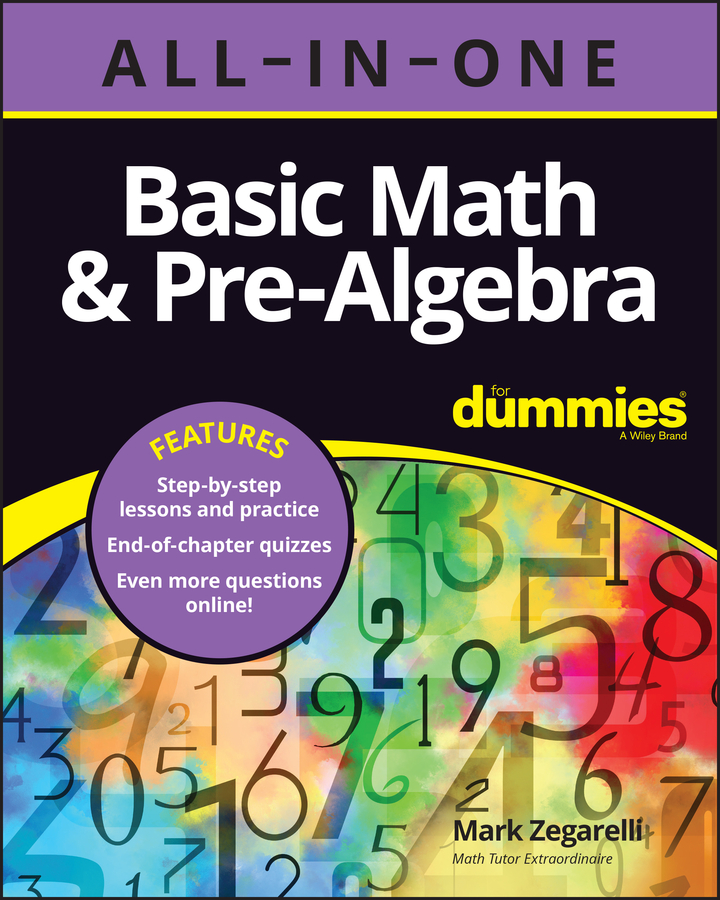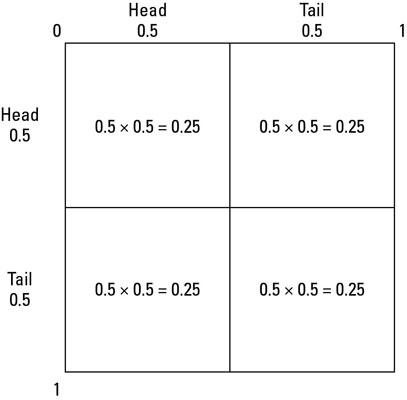##### Basic Math & Pre-Algebra All-in-One For Dummies (+ Chapter Quizzes Online)Probability squares are a really good option when you have two independent events – two things that don’t affect each other. You may like probability squares if you’re more a ‘shapes person’ than a ‘numbers person’. The probability square extends the probability number line into two dimensions by drawing two separate number lines at right angles – one line for each event.For two independent events, here’s how to use the probability square:

1. Draw a rough square.

Mark the top-left corner with 0, and the top-right and bottom-left corners both with 1. (Don’t bother with the other corner.)

2. On the horizontal probability line, mark the probability of the first event.

You don’t have to measure the line. Just put a cross somewhere that looks reasonable.

3. Draw a line down the page from your cross to the opposite edge of the square.

4. Label the horizontal probability line with the probability (to the left of the line) and the ‘what’s left over’ (one take away the probability) on the right.

5. On the vertical probability line, mark the probability of the second event.

Don’t measure – just estimate and draw a small cross.

6. Draw a line across the square from the small cross.

Your diagram should now look like a big cross. The top-left rectangle represents the probability of both events happening; the top-right rectangle shows the probability of the second event happening but not the first; the bottom-left rectangle gives you the probability of the first event happening but not the second; and the bottom-right rectangle gives you the probability of neither event happening.

7. Label the vertical line with the probability (above the line) and the number left over (below it).

8. To find the probability of both things happening – the top-left quarter – you times the number at the top of the column by the number at the left end of the row.

Remember, to get the probability of two events occurring, you have to times them together. Visually, this corresponds to the area of the rectangle, so if you were to draw it accurately the biggest areas would represent the largest probabilities.

9. To find the probability of any combination of events, find the correct area (as described in Step 6) and times the number at the top of the column by the number at the left end.